## 3.12.2023

### Sine and cosine table

This is the most unusual table of sines and cosines. The idea to make such a table came to me in the process of writing these articles:

I strongly recommend that you read these articles. Then you will know how easy it is to calculate the values of sine and cosine yourself. I made one sine and cosine table from these three articles.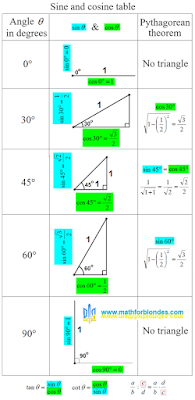Sine and cosine table

The table indicates which sides of the triangle to take as a unit. For 0 and 90 degrees, triangles do not exist, these are ordinary segments.

The third column of the table shows how to use the Pythagorean theorem to calculate the desired value of the sine or cosine. For a cosine of 30 degrees and a sine of 60 degrees, the calculations are the same.

At the bottom of the table there are hints on how to calculate the tangent and cotangent values if you know the sine and cosine values. If you forgot how to divide one fraction by another, use one more hint. You need to multiply the first fraction by the reciprocal of the second fraction.

## 3.11.2023

### Sine and cosine of 45 degrees

We have already considered what the sine of 30 degrees is and what the sine of 60 degrees is equal to. Now we will see how to find the sine and cosine of 45 degrees and why they are equal.

Last time we considered an equilateral triangle. Now we will consider an isosceles right triangle. A very rare beast in a herd of triangles. Mathematicians have known it for many thousands of years, and it is simply boring for mathematicians to tinker with it.

## Isosceles right triangle

We will consider a tired isosceles right triangle. Why is the triangle tired? He lay down on his side to rest.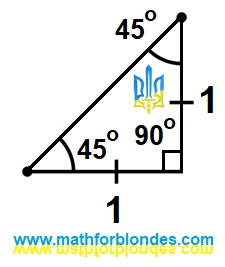Isosceles right triangle

The legs of this triangle are equal to one. Once again I repeat that both legs have the same length. We do not know the length of the hypotenuse of this triangle, but we can easily calculate it using the Pythagorean theorem.

## Sine and cosine of 45 degrees

Smart people came to us and said that the sine and cosine of an angle are equal to the ratio of the legs to the hypotenuse. Since the legs of a right triangle with an angle of 45 degrees are equal, then the value of the sine of 45 degrees is equal to the value of the cosine of 45 degrees. We take the math in hand and calculate this value.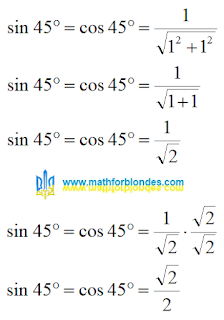Sine and cosine of 45 degrees

Why did I multiply the numerator and denominator of a fraction by the square root of two? Small children do not like lumps in porridge. Mathematicians don't like square roots in denominators. Very capricious uncles and aunts.

## 3.03.2023

### What is the sine of 60 degrees?

Before we look for the answer to the question: "What is the sine of 60 degrees?", I strongly recommend reading the post "Why is the sine of 30 degrees equal to half one?".

## Height of an equilateral triangle

I again take an equilateral triangle with a side equal to one. I draw height.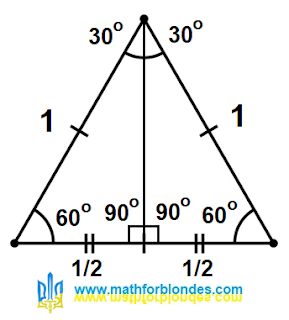Height of an equilateral triangle

After that, I will not turn anything. I'll just remove half of the drawing.

## Cosine 60 degrees

I have a right-angled triangle, the diagonal of which is equal to one.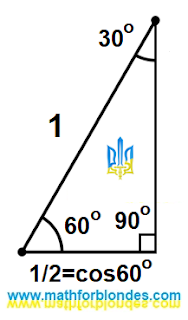Cosine 60 degrees

The base of this triangle is equal to 1/2 and is also equal to the cosine of an angle of 60 degrees.

## Sine 60 degrees

The height of this triangle is equal to the sine of the angle of 60 degrees. We calculate the height using the Pythagorean theorem.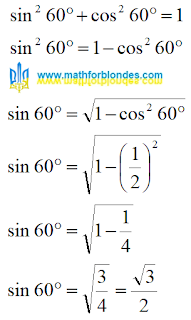Sine 60 degrees

The sine of 60 degrees is equal to the square root of three divided by two. It is calculated in the same way as the cosine of 30 degrees.

## 2.21.2023

### Where does math end?

I was offered to sell my Russian site for 45.000 rubles. This prompted me to a very interesting question: "Where does mathematics end?". And here's my answer: "Math ends where money begins". Try any mathematical theory to apply to money and you will learn a lot of interesting things about this theory.

I love math trolling. The sentence contained the phrase: "The price may be revised upwards". My response was: "\$45.000 Price may be revised down". From the point of view of mathematics, the equality 45.000=45.000 is beyond doubt. As soon as I add different units of money to this equality, it disappears.Where does math end?

We use numbers to measure money. Numbers are written on coins and banknotes. Have you heard of banknote or a coin with the number "zero"? Zero is not a number. The more I study this question, the more I am convinced of the correctness of my statement.Zero dollars

I'm not even talking about the minus sign on money. Through the prism of money, I considered set theory. The result is very interesting.

## 2.12.2023

### Why is the sine of 30 degrees equal to half one?

The answer to the question "why is the sine of 30 degrees equal to half one?" can be searched in the history of mathematics. These are ancient Mesopotamia, ancient Greece and other ancient civilizations. I am not an expert in this area. Obviously, knowledge has evolved from simpler to more complex. I will show you the most obvious answer to this question as I see it.

## Equilateral triangle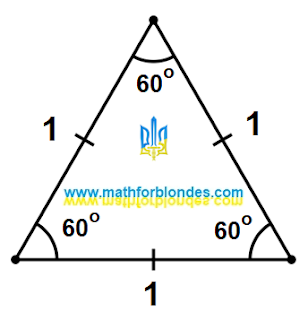Equilateral triangle

I have drawn an equilateral triangle with sides equal to one. I so want. The sum of the angles of a triangle is 180 degrees. An equilateral triangle has three 60 degree angles. I don't know trigonometry yet.

## Height of an equilateral triangleHeight of an equilateral triangle

I drew the height in an equilateral triangle. The height is always perpendicular to the base of the triangle. If the height is drawn through the vertex of such a triangle, it will divide it into two equal right-angled triangles. This is always the case in isosceles triangles. An equilateral triangle is a special case of an isosceles triangle, in which the base is equal to the sides.

What happened as a result? The height divided the angle at the apex into two equal angles 60=30+30, it divided the base into two equal segments 1=(1/2)+(1/2). I still haven't heard anything about trigonometric functions.

## Sine 30 degrees

After that, someone came up with trigonometric functions. I was told that the sine of an angle in a right triangle is the ratio of the opposite leg to the hypotenuse. How do I find the sine value for a 30 degree angle? I just flip the picture 90 degrees and remove all unnecessary.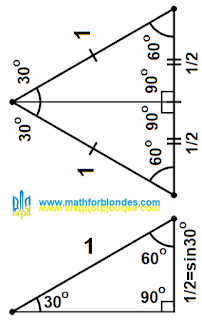Sine 30 degrees

The hypotenuse is equal to one. Any number divided by one does not change. So the length of the opposite leg in my triangle is equal to the sine of the angle of 30 degrees, that is, 1/2.

## Cosine 30 degrees

The cosine of 30 degrees I can easily find from the Pythagorean theorem. We take the Pythagorean theorem in our hands and count.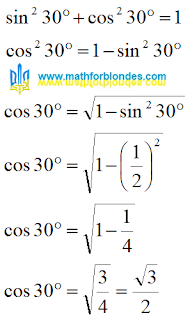Cosine 30 degrees

The cosine of 30 degrees turned out to be equal to the square root of three, divided by two.

Here's how easy it is to calculate. No tables needed.